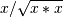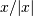# numpy.sign¶

numpy.sign(x[, out]) = <ufunc 'sign'>

Returns an element-wise indication of the sign of a number.

The sign function returns -1 if x < 0, 0 if x==0, 1 if x > 0. nan is returned for nan inputs.

For complex inputs, the sign function returns sign(x.real) + 0j if x.real != 0 else sign(x.imag) + 0j.

complex(nan, 0) is returned for complex nan inputs.

Parameters: x : array_like Input values. y : ndarray The sign of x.

Notes

There is more than one definition of sign in common use for complex numbers. The definition used here is equivalent towhich is different from a common alternative,.

Examples

>>> np.sign([-5., 4.5])
array([-1.,  1.])
>>> np.sign(0)
0
>>> np.sign(5-2j)
(1+0j)


numpy.fabs

numpy.maximum